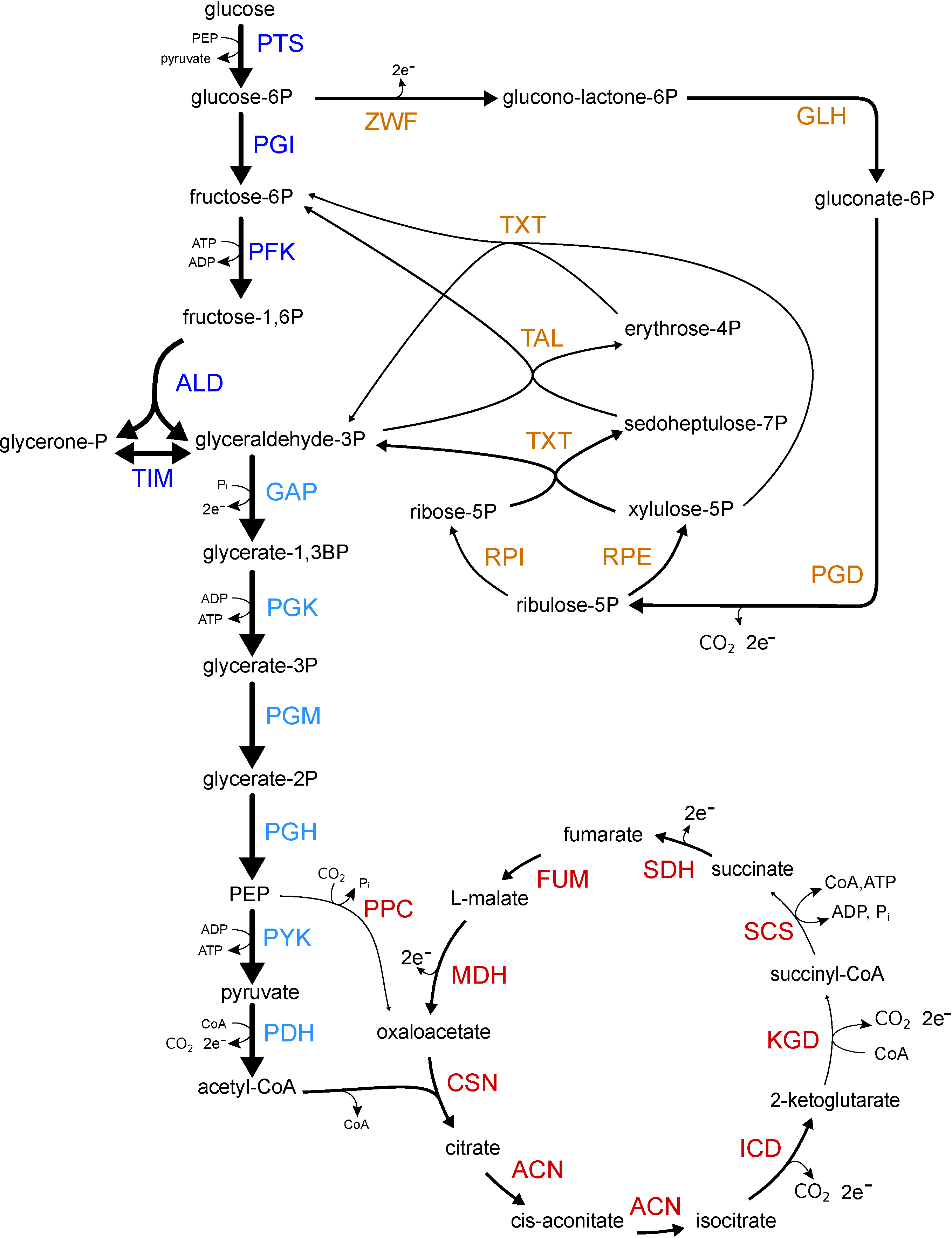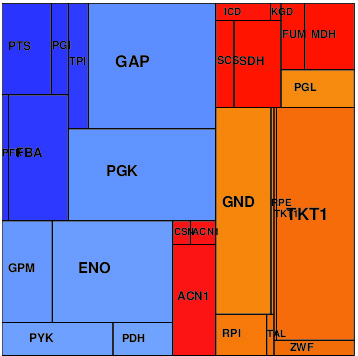# Enzyme cost minimization#### Escherichia coli model

As an example, we constructed a kinetic metabolic model of E. coli central metabolism and predict its metabolite and enzyme levels.
The kinetic constants were obtained based on known in-vitro kinetic constants by applying parameter balancing. To test the effect of protein cost weights, we ran ECM three times based on different assumptions about protein burden: uniform cost (same cost for each enzyme molecule); size-dependent cost (cost proportional to chain length); and composition-dependent cost (accounting for the different ATP investment in different amino acids). It turned out that these choices have little effect on the prediction accuracy.

#### Results

Simulation results can be found below in several data formats: in the SBtab table format (for models and data) and SBML (for models only). The NEOS files can be used for running optimizations on the NEOS Optimization Server.

 Uniform protein cost Size-dependent cost Composition-dependentResults [.pdf] Results [.pdf] Results [.pdf] Input data Input data Input data Network model [SBML] Network model [SBML] Network model [SBML] Model and data [SBtab] Model and data [SBtab] Model and data [SBtab] Model and data [NEOS files] Model and data [NEOS files] Model and data [NEOS files] Result files Result files Result files Model and EMC4cm run [SBtab] Model and EMC4cm run [SBtab] Model and EMC4cm run [SBtab] Metabolic states [SBtab] Metabolic states [SBtab] Metabolic states [SBtab]